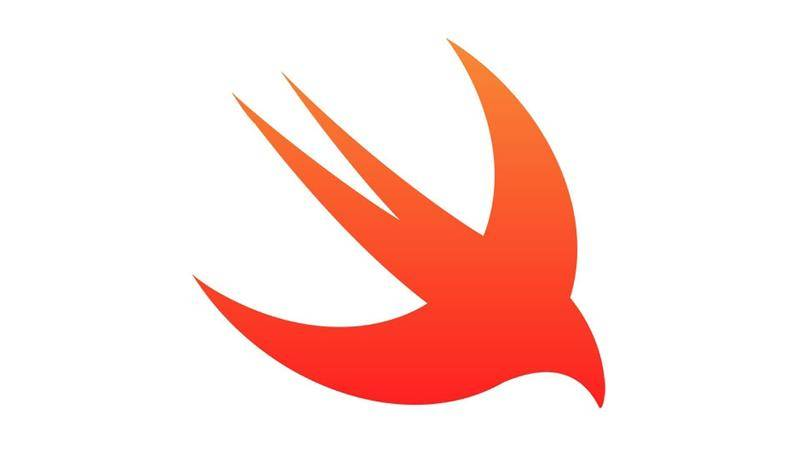### 什么是闭包？

``````//创建一个传递2个参数的闭包，并且返回值为Int类型
var add = { (a:Int,b:Int)-> Int in
return a + b
}

//还可以这样写
var add = ({ (a:Int,b:Int)-> Int in
return a + b
})

//还可以这样写
var add = (){ (a:Int,b:Int)-> Int in
return a + b
}``````

### 闭包的简写：如果闭包没有参数和返回值，那么可以删除in之前的东西（包括in）

``````var closure = { () -> Void in
print("这个是闭包")
}
//可以简写为
var closure = {
print("这个是闭包")
}``````

### 可以吧闭包当做实参传递给函数

``````func first(second: (a:Int,b:Int)->(Int)) {
print("这个是第一个方法")
let num = second(a: 10,b: 5)
print("\(num)")
}

first { (a, b) in
print("第二个方法")
return a + b
}
/* 输出结果：

15
*/``````

### 如果函数只接收一个参数，那么闭包可以直接写在()的后面

``````func add(num:Int , sub:(a:Int,b:Int) -> Int){
print("执行了add func")
let num2 = sub(a: 10,b: 5)
let result = num + num2
print("num + num2 = \(result)")
}

add(10) { (a, b) -> Int in
return a - b
}
/* 输出结果：

num + num2 = 15
*/``````

### 闭包循环引用的问题

``````
import UIKit
//使用属性来保存闭包，self.finished 保存着闭包，然后在闭包里面使用self，所以出现了循环引用
var finished:(() -> ())?

class ViewController: UIViewController {

override func viewDidLoad() {
super.viewDidLoad()
add {
print("这个在主进程")
self.view.backgroundColor = UIColor.greenColor()
}
}

func add(sub:() -> ()){
print("执行了add func")
}

deinit {
print("这里进行销毁")
}

}
``````

``````
import UIKit
//使用属性来保存闭包，self.finished 保存着闭包，然后在闭包里面使用self，所以出现了循环引用
var finished:(() -> ())?

class ViewController: UIViewController {

override func viewDidLoad() {
//把self变成弱引用即可
weak var weakSelf = self
super.viewDidLoad()
add {
print("这个在主进程")
weakSelf!.view.backgroundColor = UIColor.greenColor()
}
}

func add(sub:() -> ()){
print("执行了add func")
}

}``````